### IMO Shortlist 2014 problem G3

Kvaliteta:
Avg: 4,0
Težina:
Avg: 7,0

Let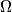$\Omega$ and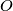$O$ be the circumcircle and the circumcentre of an acute-angled triangle$ABC$ with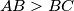$AB > BC$. The angle bisector of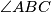$\angle ABC$ intersects$\Omega$ at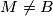$M \neq B$. Let$\Gamma$ be the circle with diameter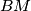$BM$. The angle bisectors of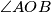$\angle AOB$ and$\angle BOC$ intersect$\Gamma$ at points$P$ and$Q$, respectively. The point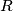$R$ is chosen on the line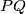$PQ$ so that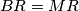$BR = MR$. Prove that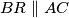$BR \parallel AC$. (Here we always assume that an angle bisector is a ray.)

(Russia)

Izvor: https://www.imo-official.org/problems/IMO2014SL.pdf